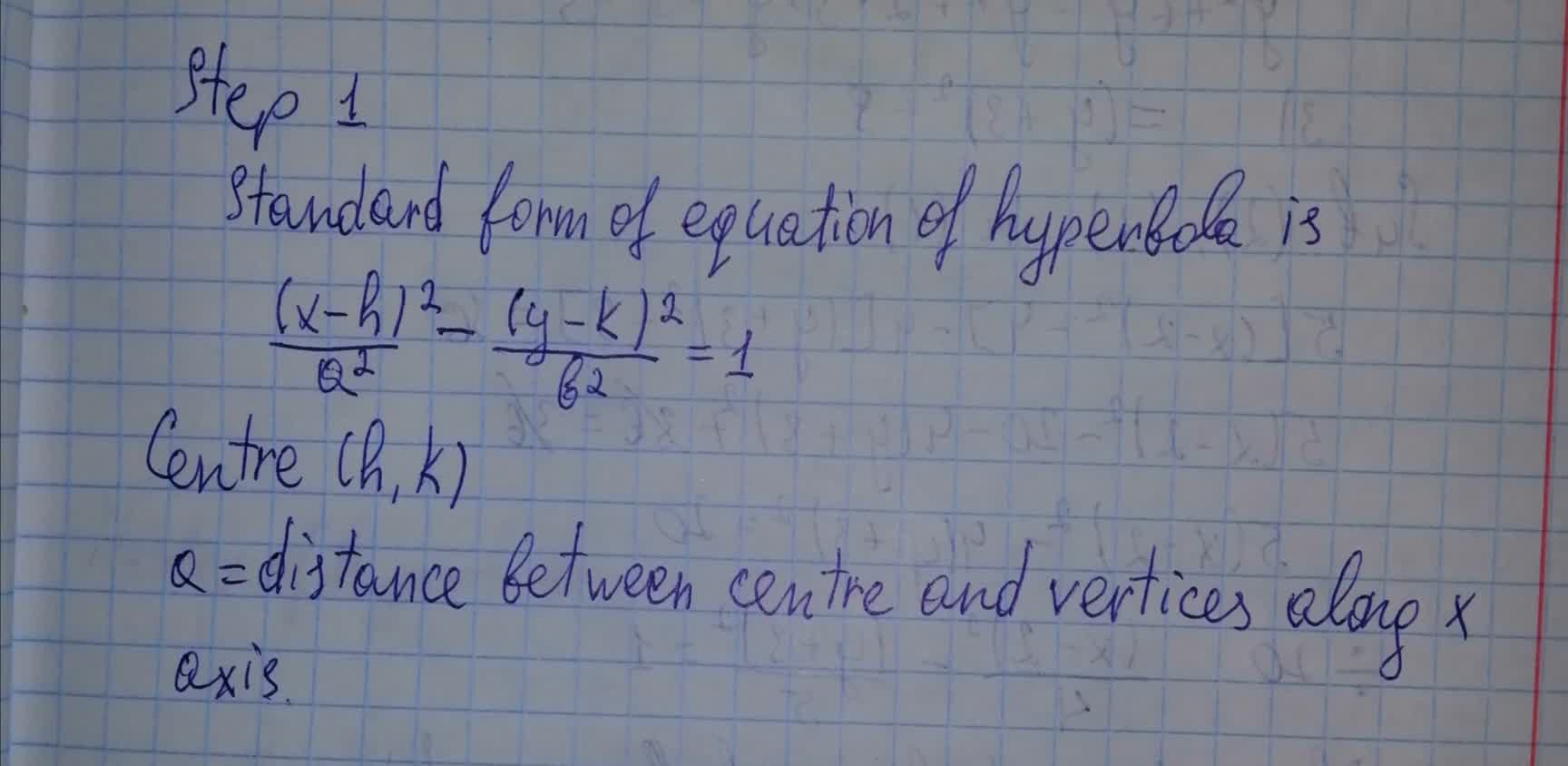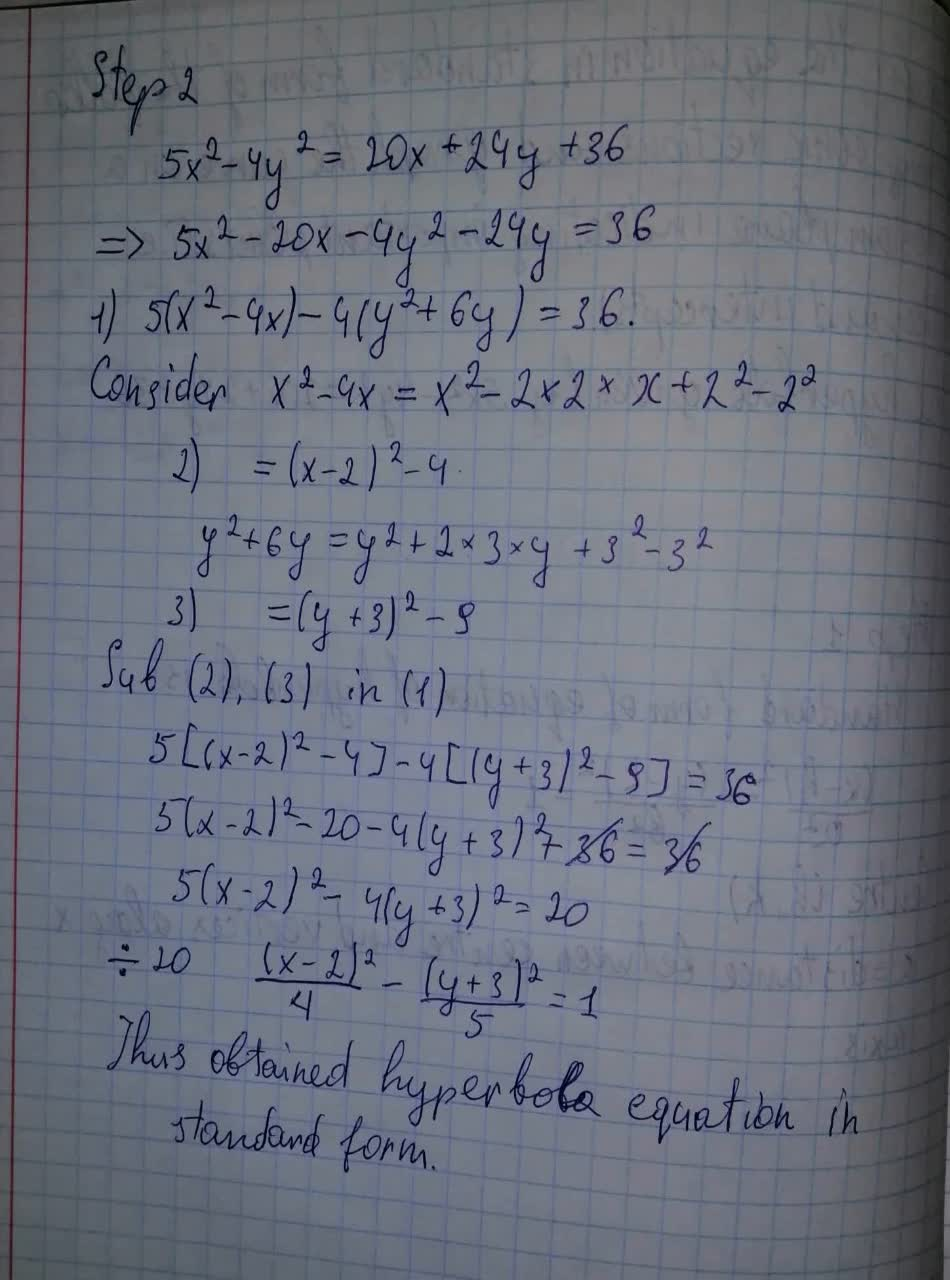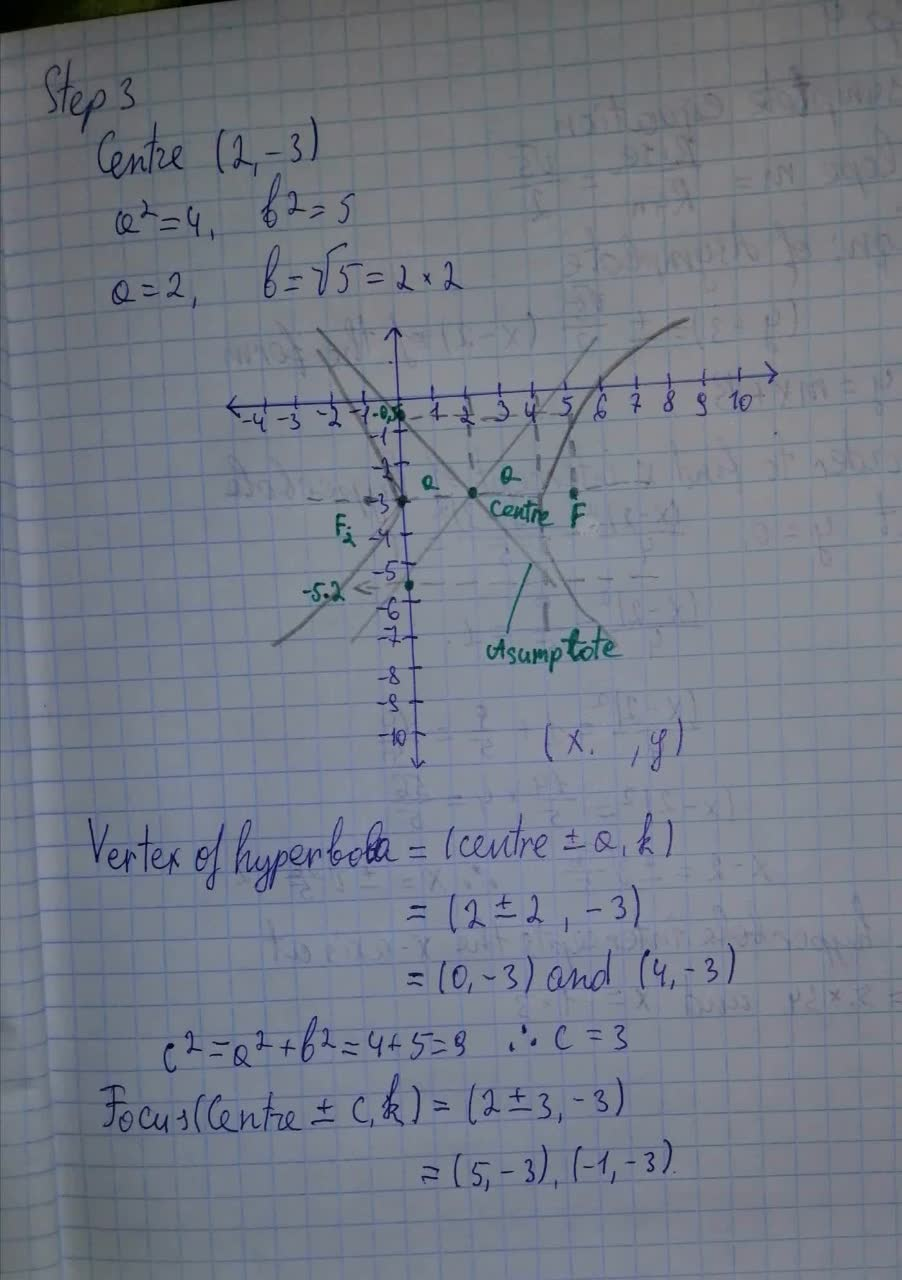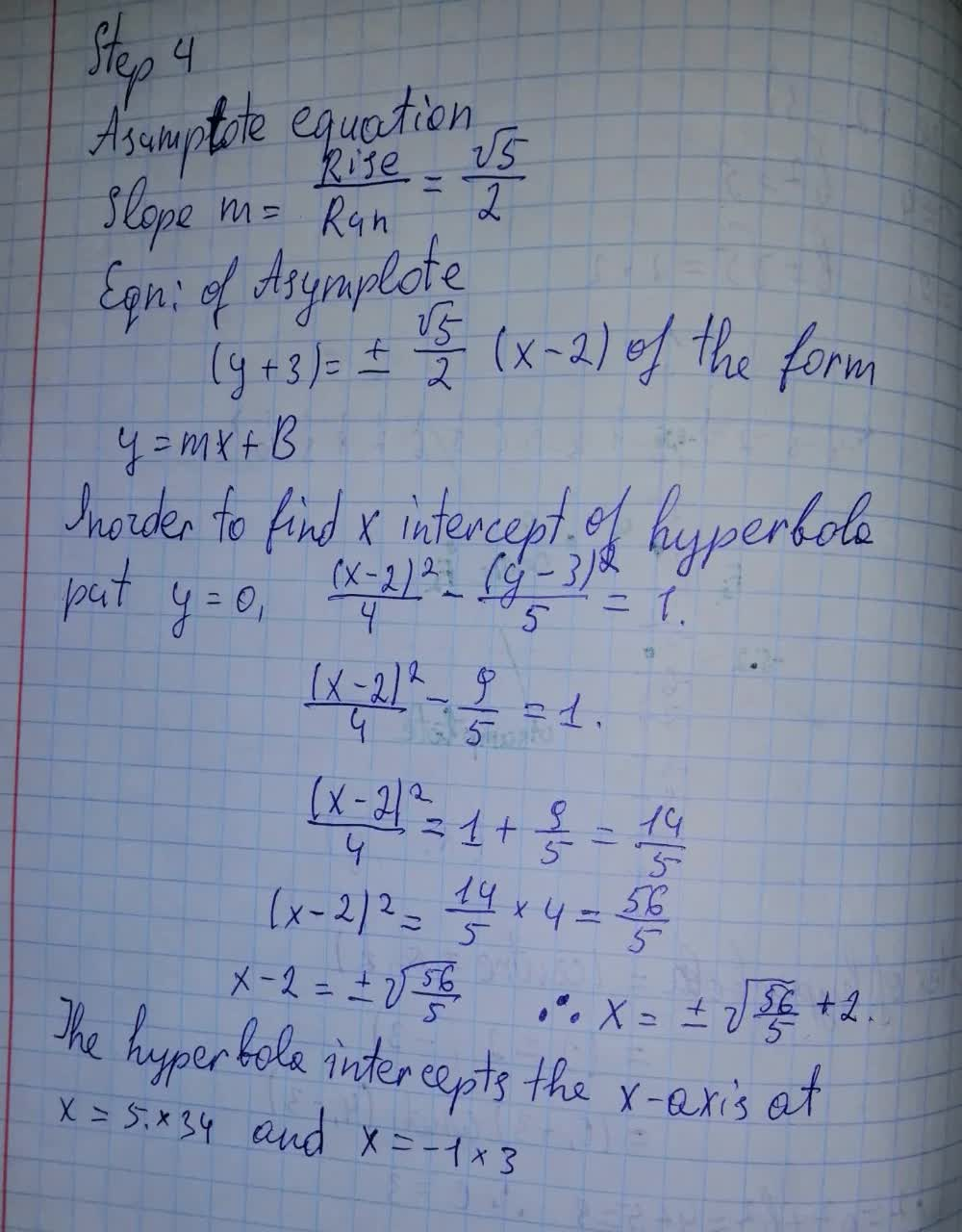# The hyperbola given by 5x^2 – 4y^2= 20x + 24y + 36abondantQ 2021-08-12 Answered
Solve for the equation in standard form of the following conic sections and graph the curve on a Cartesian plane indicating important points (i.e. vertices and intercepts).The hyperbola given by $$\displaystyle{5}{x}^{{2}}–{4}{y}^{{2}}={20}{x}+{24}{y}+{36}$$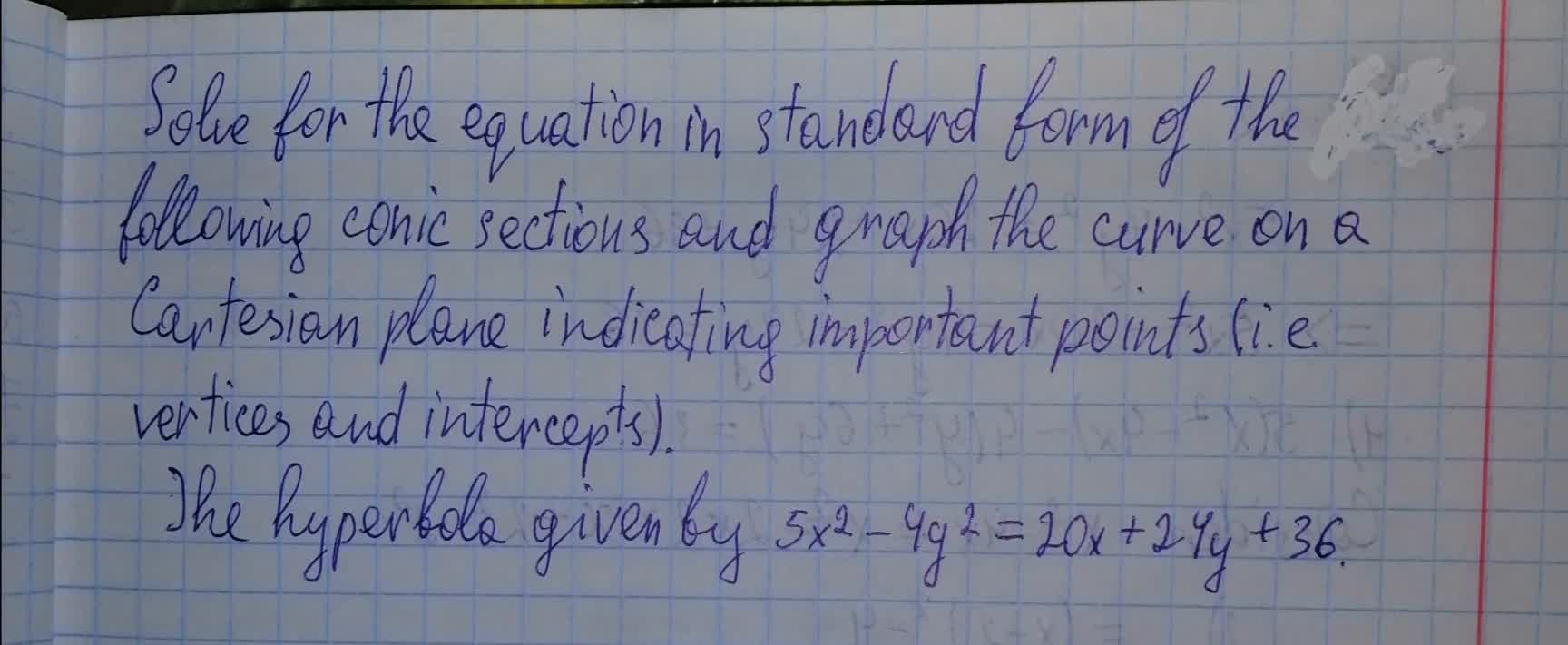• Questions are typically answered in as fast as 30 minutes

### Plainmath recommends

• Get a detailed answer even on the hardest topics.
• Ask an expert for a step-by-step guidance to learn to do it yourself.Bella

Solved it in photo below